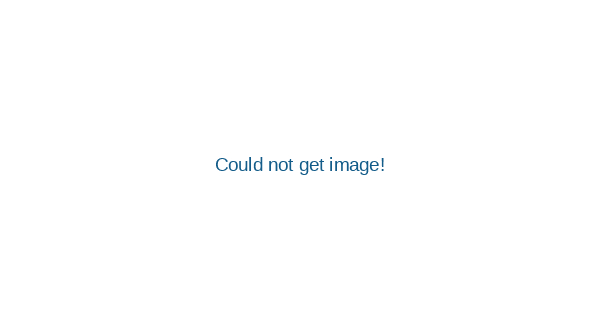VLOOKUP is a very commonly used formula within business.  Once you realise how powerful it can be, you will begin using it for more and more time-saving exercises that would have otherwise taken ten times longer.  The formula is exactly the same in all versions of Excel and with Google Sheets; these screenshots are from Excel 2013 version.

In this example we have a column of reference codes in Column A, and the associated addresses in Column B.  We need to return the correct address when we type in the reference code into cell C1.VLOOKUP has 4 “arguments” (parts that need to be filled in to make the formula work, separated by commas), these are: lookup value, table array, column index, and range lookup.In this example, we want the result to appear in cell D1.  So begin by clicking in cell D1 and and typing:

=VLOOKUP(

Excel will then give you a handy “tooltip” to remind you what information it needs to make this formula work.  The first thing it needs is the “lookup_value”; this is the bit of information you already have (in our case the reference code) that you will be looking up against.  Our lookup value (the reference code) is in cell C1, so either type C1 or click in cell C1 and excel will fill that in for you.  Next type a comma, so now you have

=VLOOKUP(C1,The tooltip will change to embolden the text “table_array” as a reminder that you now need to tell it which range of cells you need to lookup in.  In our example, the data begins in cell A1, and extends to B10 (all the reference codes and addresses).  To enter this range, either type in A1:B10 or simply use your mouse to draw a box around cells A1 to B10 and excel will enter this range into the formula.  Again, type a comma before moving onto the next part of the formula:

=VLOOKUP(C1,A1:B10,

Next we have “col_index_num”, which is telling the formula which column we want to return the data from.   Our example only has data in two columns (A and B), the first column being the reference codes and the 2nd being the addresses.  We want the info from the 2nd column to be returned so for this section we type 2 and another comma.

=VLOOKUP(C1,A1:B10,2,

For the last part (“range_lookup”) we have the option of returning either only exact matches, or the closest match (if the data is sorted in order).  There are very few situations where you will not want an exact match.  To instruct the formula to only return an exact match, type FALSE or for the closest match (if the data is in sorted order…) then type TRUE.  Get used to always typing in FALSE for this section and you won’t go far wrong.  To complete the formula type a closed bracket.  The completed formula:

=VLOOKUP(C1,A1:B10,2,FALSE)

All has gone well and the formula has worked as expected; reference code 2295031 has been found within the data and returned the corresponding address which is 18 Steward Way.